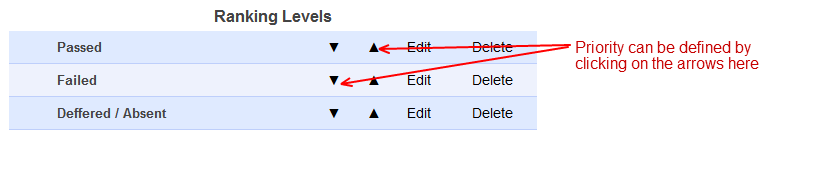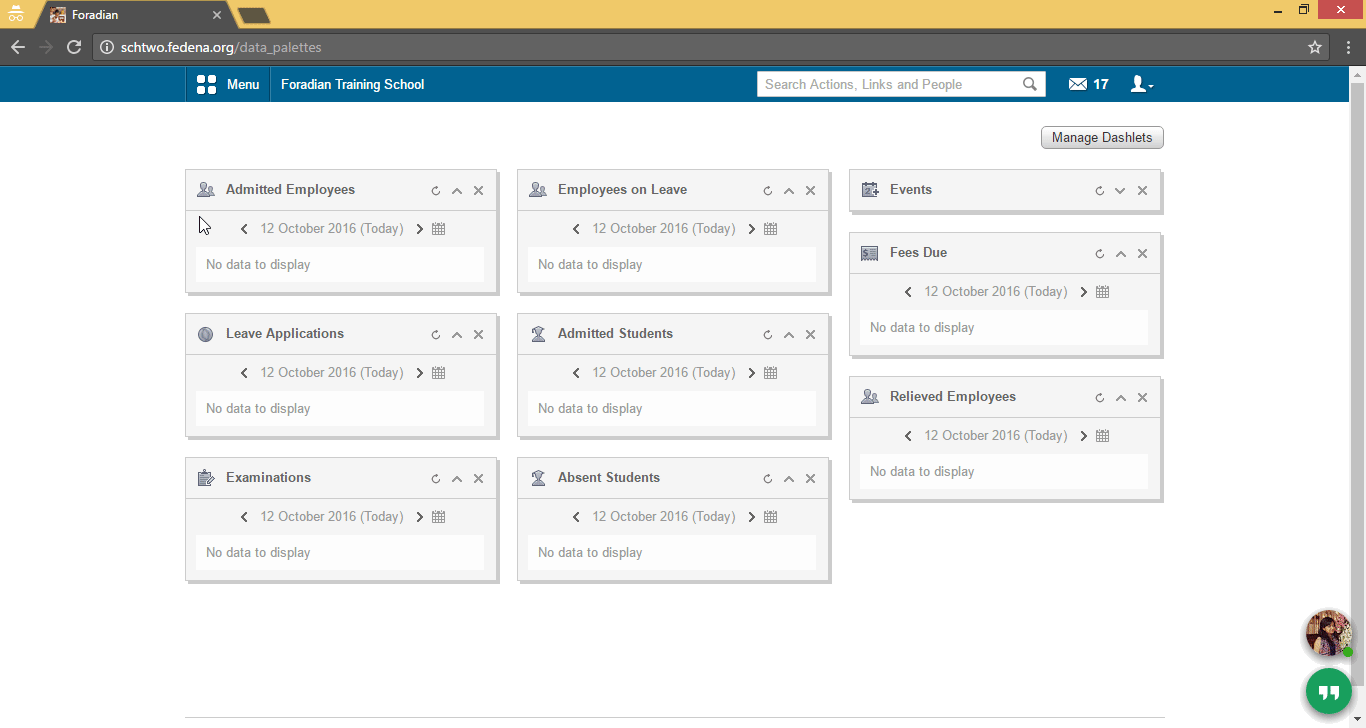There is an option todefine the priority levels of the Ranking levels created. This is extremelyimportant. If a student falls in two criteria (Say the student is passed andhas got a first class, then normally the student should be listed in the firstclass list. So that Ranking level should be given more priority), the studentwill be listed in the Ranking level which is defined to have the higherpriority

Eg: Student got 85%marks, suppose as per the Ranking levels defined, he falls in two list, Say 1)First Class with criteria as minimum 80% marks in at least 4 subjects & 2)Second Class with criteria as minimum 70% in at least 4 subjects. Now as per themarks the student got, he/she is eligible to be included in the first list,i.e, list of students having first class. So that Ranking level should be givenhighest priority.Priority list can be easily edited by clicking on the arrows. TheRanking level with highest priority is will be shown at the top and the nextone below and so on.

Some examples of Ranking Level:

Referred ranking level

Let GPA level is set as ‘2.50’ and the GPAlimit type is ‘Upper’ means the student has the GPA level below ‘2.50’. Letnumber of subjects is given as ‘3’ and subject limit type is given as ‘upper’means the student has got less than 3 subjects with GPA below 2.5 and falls in‘Referred’ Ranking level

Failedranking level

Let the GPA level is ‘2.50’ and the GPA limittype is ‘Upper’ means the students have GPA level below ‘2.50’. Let number ofsubjects s given as ‘3’ and the subject limit type is given as ‘lower’ meansthe students has failed in more than 3 subjects and falls in ‘Failed’ Rankinglevel

Absent ranking level

Let the GPA level is ‘0’ and the GPA limittype is ‘Exact’ means the student have the GPA level equal to ‘0’ not more thanor less than ‘0’. Let the number of subject is ‘1’ and the subject limit typeis ‘lower’ means the student is absent in more than one subject and the studentfalls in the ‘Absent’ Ranking level

Passed ranking level

Let the GPA level is ‘2.50’ and the GPA limittype is ‘lower’ means the student have GPA level more than ‘2.50’. Let thenumber of subjects is 6 and the subject limit type is ‘Exact’ means thestudents has to get more than 2.5 GPA in exactly 6 subjects to fall in to‘Passed’ Ranking level category

First Class ranking level

Letthe GPA level is ‘4.0’ and the GPA limit type is lower means the student shouldhave GPA level more than ‘4’. Let the number of subjects is not given and thesubject limit type is not given, This means that irrespective of the GPA inindividual subject, if the total GPA of the student is above 4, that studentfalls in First Class Ranking level.